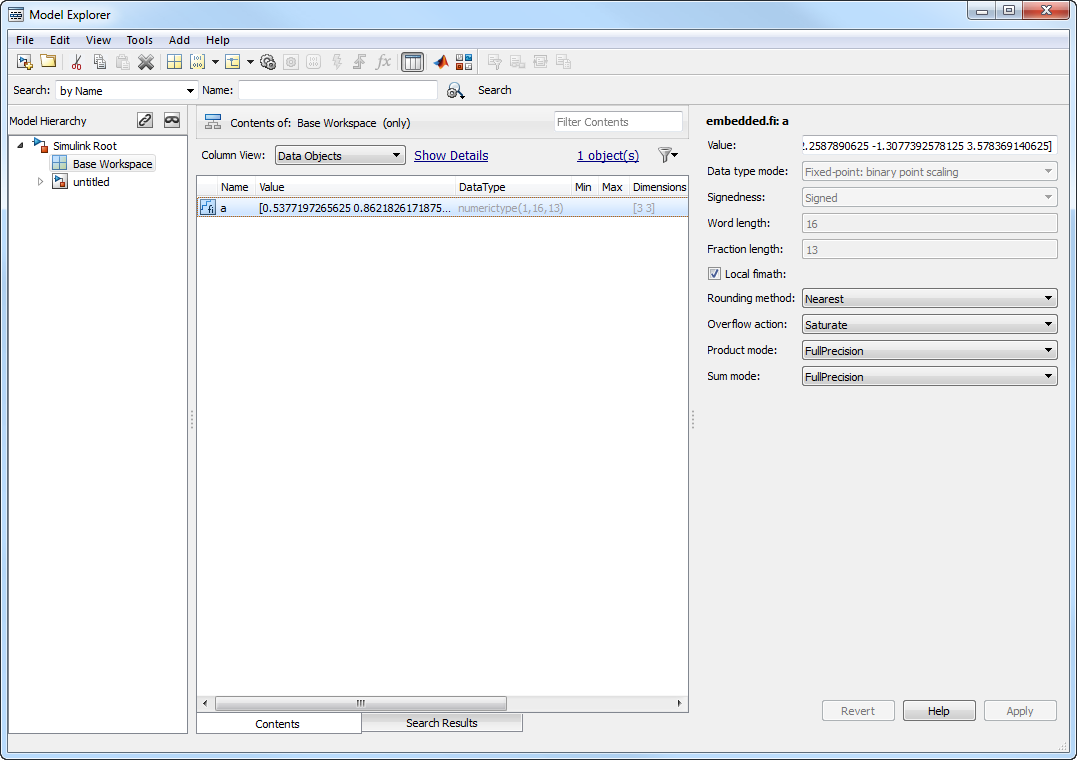### View and Edit `fi` objects in Model Explorer

You can view and edit `fi` objects and their local `fimath` properties using Model Explorer in Simulink®. You can change the writable properties of `fi` objects from the Model Explorer, but you cannot change the numeric type properties of `fi` objects after creation.### Reading Fixed-Point Data from the Workspace

You can read fixed-point data from the MATLAB® workspace into a Simulink model via the From Workspace block. To do so, the data must be in a structure format with a `fi` object in the `values` field. In array format, the From Workspace block only accepts real, double-precision data.

To read in `fi` data, the Interpolate data parameter of the From Workspace block must not be selected, and the Form output after final data value by parameter must be set to anything other than `Extrapolation`.

### Writing Fixed-Point Data to the Workspace

You can write fixed-point output from a model to the MATLAB workspace via the To Workspace block in either array or structure format. Fixed-point data written by a To Workspace block to the workspace in structure format can be read back into a Simulink model in structure format by a From Workspace block.

### Note

To write fixed-point data to the MATLAB workspace as a `fi` object, select the Log fixed-point data as a fi object check box on the To Workspace block dialog. Otherwise, fixed-point data is converted to `double` and written to the workspace as `double`.

For example, you can use the following code to create a structure in the MATLAB workspace with a `fi` object in the `values` field. You can then use the From Workspace block to bring the data into a Simulink model.

```a = fi([sin(0:10)' sin(10:-1:0)']) a = 0 -0.5440 0.8415 0.4121 0.9093 0.9893 0.1411 0.6570 -0.7568 -0.2794 -0.9589 -0.9589 -0.2794 -0.7568 0.6570 0.1411 0.9893 0.9093 0.4121 0.8415 -0.5440 0 DataTypeMode: Fixed-point: binary point scaling Signedness: Signed WordLength: 16 FractionLength: 15 s.signals.values = a s = signals: [1x1 struct] s.signals.dimensions = 2 s = signals: [1x1 struct] s.time = [0:10]' s = signals: [1x1 struct] time: [11x1 double] ```

The From Workspace block in the following model has the `fi` structure `s` in the Data parameter.

Remember, to write fixed-point data to the MATLAB workspace as a `fi` object, select the Log fixed-point data as a fi object check box on the To Workspace block dialog. Otherwise, fixed-point data is converted to `double` and written to the workspace as `double`.

In the model, the following parameters in the Solver pane of the Model Configuration Parameters dialog have the indicated settings:

• Start time`0.0`

• Stop time`10.0`

• Type`Fixed-step`

• Solver```Discrete (no continuous states)```

• Fixed step size (fundamental sample time)`1.0`The To Workspace block writes the result of the simulation to the MATLAB workspace as a `fi` structure.

```simout.signals.values ans = 0 -8.7041 13.4634 6.5938 14.5488 15.8296 2.2578 10.5117 -12.1089 -4.4707 -15.3428 -15.3428 -4.4707 -12.1089 10.5117 2.2578 15.8296 14.5488 6.5938 13.4634 -8.7041 0 DataTypeMode: Fixed-point: binary point scaling Signedness: Signed WordLength: 32 FractionLength: 25 ```

### Setting the Value and Data Type of Block Parameters

You can use Fixed-Point Designer™ expressions to specify the value and data type of block parameters in Simulink. For more information, see Specify Fixed-Point Data Types (Simulink).

### Logging Fixed-Point Signals

When fixed-point signals are logged to the MATLAB workspace via signal logging, they are always logged as `fi` objects.

To enable signal logging for a signal:

1. Select the signal.

2. Open the Record dropdown.

3. Select Log/Unlog Selected Signals.

When you log signals from a referenced model or Stateflow® chart in your model, the word lengths of `fi` objects may be larger than you expect. The word lengths of fixed-point signals in referenced models and Stateflow charts are logged as the next largest data storage container size.

### Accessing Fixed-Point Block Data During Simulation

Simulink provides an application program interface (API) that enables programmatic access to block data, such as block inputs and outputs, parameters, states, and work vectors, while a simulation is running. You can use this interface to develop MATLAB programs capable of accessing block data while a simulation is running or to access the data from the MATLAB command line. Fixed-point signal information is returned to you via this API as `fi` objects. For more information on the API, refer to “Accessing Block Data During Simulation” in the Simulink documentation.

##### SupportGet trial now